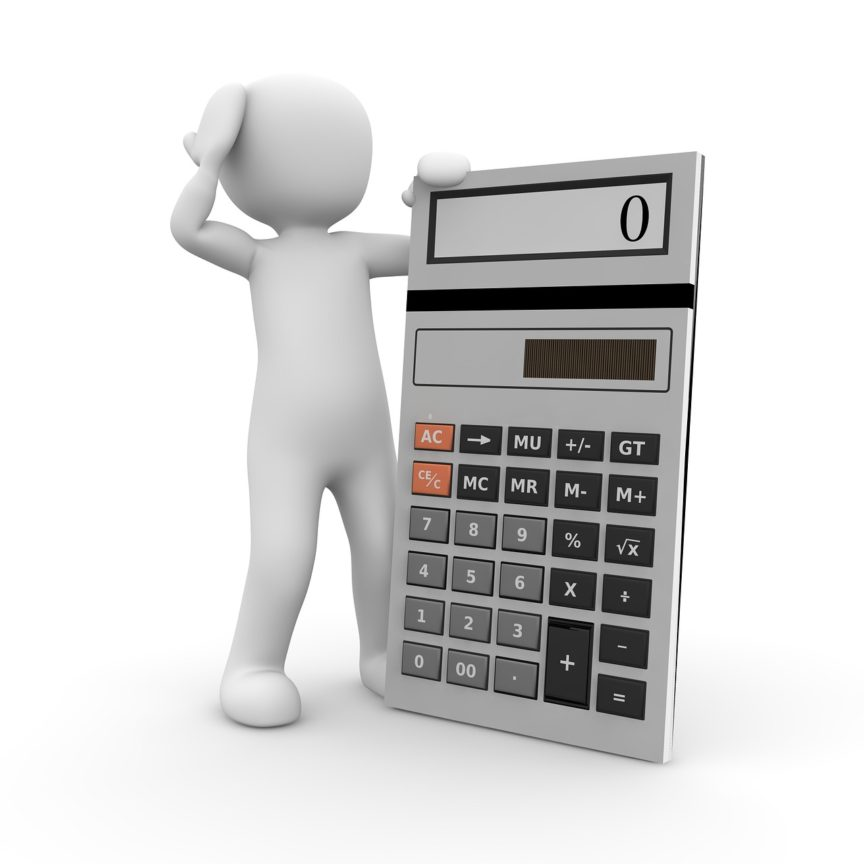# Calculating the SBA Guaranty Fee 2022

In SBA Loans by Key Commercial Capital

## The following are instructions on how to calculate the SBA Guaranty Fee at different loan amounts.

Depending on the loan amount, the SBA has different ways to calculate the fee amount. Examples are included for each level.

### Loan Amount:  50,000 to 350,000

ZERO (0%) – Currently there is not an SBA Guaranty Fee for loans under 350,000.

It is being waived.  In the past it was as follows in the example below.  This will be updated as changes occur in the SBA Standard Operating Procedures.

#### Example 150,000 x .85 = 127,500 x .02 = 2,550 is the SBA Guaranty Fee

————————————————————

### Loan Amount:  350,001 to 700,000

Take loan amount and multiply by .75

Then take that figure and multiply by .0277 (2.77%)

#### Example 350,001 x .75 = 262,500.75 x .0277 = 7,271.27 is the SBA Guaranty Fee

————————————————————

### Loan Amount:  700,001 to 1,000,000

Take loan amount and multiply by .75

Then take that figure and multiply by .0327 (3.27%)

#### Example 700,001 x .75 = 525,000.75 x .0327 = 17,167.52 is the SBA Guaranty Fee

————————————————————

### Loan Amount: 1,000,001 to 5,000,000

This one is 3 steps

Example 3,250,000 would be figured as such

Step 1 to figure fee for 1,000,000

Take loan amount of 1,000,000 and multiply x .75 = 750,000 x .0327 (3.27%) = 26,250

Step 2 to figure fee for amount over 1,000,000 in this case 2,250,000

2,250,000 (amount over 1MM) x .75 = 1,687,500 x .0375 (3.75%) = 63,281.25

#### 63,281.25 + 26,250 =  89,531.25 is the SBA Guaranty Fee

————————————————————

If you have further questions about SBA guarantee fees or the loan process.  Feel free to contact us for a free consultation.

What are the benefits of working with a business loan broker or business loan brokerage firm to find financing?